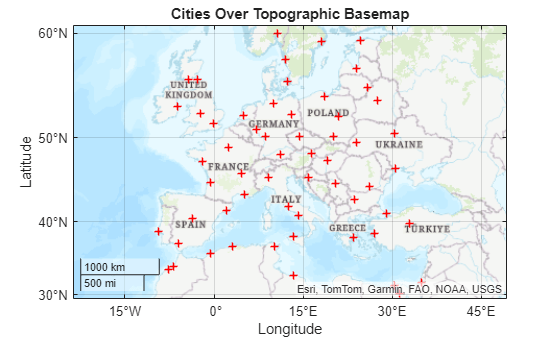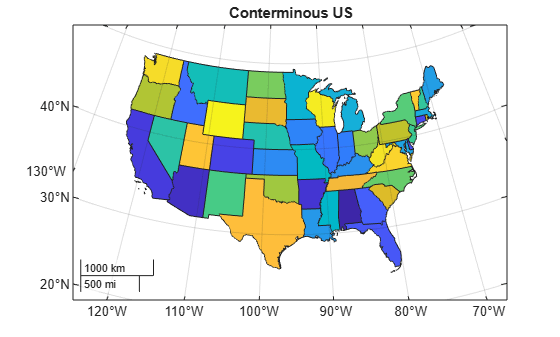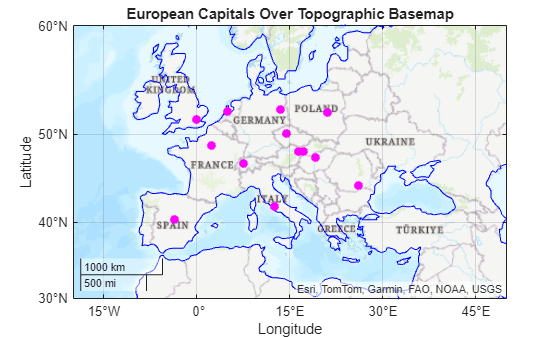# geoplot

Plot points, lines, and polygons in geographic axes

## Syntax

``geoplot(GT)``
``geoplot(shape)``
``geoplot(___,LineSpec)``
``geoplot(lat,lon)``
``geoplot(lat,lon,LineSpec)``
``geoplot(lat1,lon1,...,latN,lonN)``
``geoplot(lat1,lon1,LineSpec1,...,latN,lonN,LineSpecN)``
``geoplot(tbl,latvar,lonvar)``
``geoplot(___,Name=Value)``
``geoplot(gx,___)``
``h = geoplot(___)``

## Description

Mapping Toolbox™ extends the functionality of the `geoplot` (MATLAB®) function. It adds support for displaying points, lines, and polygons with coordinates in any supported geographic or projected coordinate reference system (CRS). If you do not have Mapping Toolbox installed, then see `geoplot`.

### Geospatial Table and Shape Data

example

````geoplot(GT)` plots the point, line, or polygon shape objects within the `Shape` variable of the geospatial table `GT`.```

example

````geoplot(shape)` plots the point, line, or polygon shape objects in `shape` on a geographic axes.```

example

````geoplot(___,LineSpec)` sets the style, marker symbol, and color for the plotted shapes. You can use the `LineSpec` argument with either of the `GT` or `shape` arguments.```

### Numeric Vector Data

````geoplot(lat,lon)` plots a line with vertices at the latitudes and longitudes in `lat` and `lon`, respectively.```

example

````geoplot(lat,lon,LineSpec)` sets the style, marker symbol, and color for the line.```
````geoplot(lat1,lon1,...,latN,lonN)` plots multiple pairs of latitude and longitude coordinates on the same set of geographic axes.```
````geoplot(lat1,lon1,LineSpec1,...,latN,lonN,LineSpecN)` assigns specific line styles, markers, and colors to each latitude-longitude pair.```

### Numeric Table Data

````geoplot(tbl,latvar,lonvar)` plots the variables `latvar` and `lonvar` from the table `tbl`. To plot one data set, specify one variable for `latvar` and one variable for `lonvar`. To plot multiple data sets, specify multiple variables for `latvar`, `lonvar`, or both. If both arguments specify multiple variables, they must specify the same number of variables. (Since R2022b)```

example

````geoplot(___,Name=Value)` specifies options for the plot using one or more name-value arguments.```

example

````geoplot(gx,___)` plots in the geographic axes specified by `gx` instead of the current axes. ```

example

````h = geoplot(___)` returns a `Point`, `Line`, or `Polygon` object. The type of object depends on the type of input. Use `h` to modify the properties of the plot object.```

## Examples

collapse all

Import a shapefile containing road data for Concord, MA, into the workspace as a geospatial table. The table represents roads using line shapes in projected coordinates.

```GT = readgeotable("concord_roads.shp"); GT.Shape```
```ans=609×1 object 16×1 maplineshape array with properties: NumParts: [16×1 double] Geometry: "line" CoordinateSystemType: "planar" ProjectedCRS: [1×1 projcrs] ⋮ ```

Display the line shapes by passing the table to the `geoplot` function.

```figure geoplot(GT)```

Change the basemap and add a title.

```geobasemap streets title("Road Network Over Streets Basemap")```One way to plot data from a geospatial table and customize the colors is to set the `ColorVariable` property. You can set this property by using a name-value argument when you call the `geoplot` function, or you can set it on the plot object later.

Read a shapefile containing tsunami (tidal wave) events into the workspace as a geospatial table. The table represents the tsunami events using point shapes in geographic coordinates.

`GT = readgeotable("tsunamis.shp",CoordinateSystemType="geographic");`

Create a subtable containing events for a region surrounding Southeast Asia.

```bbox = geopolyshape([-25 35 35 -25 -25],[90 90 170 170 90]); inpoly = isinterior(bbox,GT.Shape); GT2 = GT(inpoly,:);```

Display the point shapes within the table. Vary the marker colors by specifying the `ColorVariable` name-value argument as a table variable. Return the `Point` object as `h`, so you can change the `ColorVariable` property later.

```figure h = geoplot(GT2,ColorVariable="Year",MarkerSize=20);```

```geobasemap grayterrain colorbar title("Tsunamis by Year")```Change the marker colors again by setting the `ColorVariable` property to a different table variable.

```h.ColorVariable = "Max_Height"; title("Tsunamis by Maximum Height")```Read a shapefile containing world cities into the workspace as a geospatial table. The table represents the cities using point shapes in geographic coordinates.

```GT = readgeotable("worldcities.shp"); shape = GT.Shape```
```shape = 318×1 geopointshape array with properties: NumPoints: [318×1 double] Latitude: [318×1 double] Longitude: [318×1 double] Geometry: "point" CoordinateSystemType: "geographic" GeographicCRS: [1×1 geocrs] ```

Clip the shapes to a region containing part of Europe.

`clipped = geoclip(shape,[30 60],[-20 35]);`

Display the shapes using red plus sign markers over a topographic basemap.

```figure geoplot(clipped,"r+") geobasemap topographic title("Cities Over Topographic Basemap")```Read hydrography data into the workspace as a geospatial table. The table represents the data using polygon shapes in projected coordinates. Extract the polygon shape for a pond.

```GT = readgeotable("concord_hydro_area.shp"); shape = GT.Shape(14)```
```shape = mappolyshape with properties: NumRegions: 1 NumHoles: 3 Geometry: "polygon" CoordinateSystemType: "planar" ProjectedCRS: [1×1 projcrs] ```

To plot shapes in projected coordinates using the `geoplot` function, the `ProjectedCRS` property of the shape must not be empty. View the contents of the `ProjectedCRS` property.

`shape.ProjectedCRS`
```ans = projcrs with properties: Name: "NAD83 / Massachusetts Mainland" GeographicCRS: [1×1 geocrs] ProjectionMethod: "Lambert Conic Conformal (2SP)" LengthUnit: "meter" ProjectionParameters: [1×1 map.crs.ProjectionParameters] ```

Display the polygon shape. Then, change the basemap and add a title.

```figure geoplot(shape) geobasemap streets title("Pond Over Streets Basemap")```Read a shapefile of US states into the workspace as a geospatial table. The table represents the states using polygon shapes in geographic coordinates.

```GT = readgeotable("usastatehi.shp"); shape = GT.Shape;```

Clip the shapes to a region containing the conterminous US.

`clipped = geoclip(shape,[17 56],[-127 -65]);`

Display the shapes. Vary the colors by using the `ColorData` name-value argument.

```figure c = 1:length(clipped); geoplot(clipped,ColorData=c)```

Change the basemap and add a title.

```geobasemap grayland title("Conterminous US")```Load a MAT file containing the coordinates of global coastlines into the workspace. The variables within the MAT file, `coastlat` and `coastlon`, specify numeric latitude and longitude coordinates, respectively. Display the coordinates using a blue line over a topographic basemap.

```load coastlines figure geoplot(coastlat,coastlon,"b") geobasemap topographic```

Read the geographic coordinates of European capitals into the workspace. Display the capitals using magenta circle markers on the same map.

```[lat,lon] = readvars("european_capitals.txt"); hold on geoplot(lat,lon,"om",MarkerFaceColor="m") title("European Capitals Over Topographic Basemap")```

Center the map over Europe by changing its limits.

`geolimits([30 60],[-13 43])`Import several shapefiles into the workspace as geospatial tables.

• `landareas.shp` contains world land areas. The table represents the areas using polygon shapes in geographic coordinates (`geopolyshape` objects).

• `worldrivers.shp` contains world rivers. The table represents the rivers using line shapes in geographic coordinates (`geolineshape` objects).

• `worldcities.shp` contains world cities. The table represents the cities using point shapes in geographic coordinates (`geopointshape` objects).

```land = readgeotable("landareas.shp"); rivers = readgeotable("worldrivers.shp"); cities = readgeotable("worldcities.shp");```

Display each set of shapes using separate calls to the `geoplot` function. Different shapes support different name-value arguments.

• Display the land areas using green polygons.

• Display the rivers using blue lines.

• Display the cities using black points.

```figure geoplot(land,FaceColor=[0.7 0.9 0.5],EdgeColor=[0.7 0.9 0.5]) hold on geoplot(rivers,Color=[0 0.4470 0.7410]) geoplot(cities,"k")```

Remove the basemap and add a title. Then, zoom in by changing the geographic limits.

```geobasemap none title("World Land Areas, Rivers, and Cities") geolimits([-72 85],[-180 180])```Create multiple geographic axes in a single figure by using a tiled chart layout.

Create a 1-by-2 tiled chart layout.

`t = tiledlayout(1,2);`

Load a MAT file containing the coordinates of global coastlines into the workspace. The variables within the MAT file, `coastlat` and `coastlon`, specify numeric latitude and longitude coordinates, respectively.

`load coastlines`

Place a geographic axes in the first tile. Plot the coordinates as a line on the axes.

```gx1 = geoaxes(t); geoplot(gx1,coastlat,coastlon) title(gx1,"Coastline Coordinates")```

Read a shapefile containing world land areas into the workspace as a geospatial table. The table represents the land areas using polygon shapes in geographic coordinates.

`GT = readgeotable("landareas.shp");`

Place a new geographic axes in the second tile. Plot the land areas as a polygon on the new axes.

```gx2 = geoaxes(t); gx2.Layout.Tile = 2; geoplot(gx2,GT) title(gx2,"Land Areas")```

Zoom both axes to a region containing Africa.

```latlim = [-55 60]; lonlim = [-21 53]; geolimits(gx1,latlim,lonlim) geolimits(gx2,latlim,lonlim)```Import several shapefiles into the workspace as geospatial tables.

• `landareas.shp` contains world land areas. The table represents the areas using polygon shapes in geographic coordinates (`geopolyshape` objects).

• `worldrivers.shp` contains world rivers. The table represents the rivers using line shapes in geographic coordinates (`geolineshape` objects).

• `worldcities.shp` contains world cities. The table represents the cities using point shapes in geographic coordinates (`geopointshape` objects).

```land = readgeotable("landareas.shp"); rivers = readgeotable("worldrivers.shp"); cities = readgeotable("worldcities.shp");```

Display each set of shapes by using separate calls to the `geoplot` function.

• Display the land areas and return the polygon in `h1`. The polygon in `h1` represents multiple polygon shapes in `land`.

• Display the rivers and return the line in `h2`. The line in `h2` represents multiple line shapes in `rivers`.

• Display the cities and return the point in `h3`. The point in `h3` represents multiple point shapes in `cities`.

```figure h1 = geoplot(land); hold on h2 = geoplot(rivers); h3 = geoplot(cities);```

Change the geographic limits of the map, add a title, and remove the basemap.

```geolimits([-72 85],[-180 180]) title("World Land Areas, Rivers, and Cities") geobasemap none```Update properties of the polygon, point, and line objects. Each object supports different properties.

• Change the fill and outline colors of the polygons to green.

• Change the color of the lines to blue.

• Change the color of the markers to black.

```h1.FaceColor = [0.7 0.9 0.5]; h1.EdgeColor = [0.7 0.9 0.5]; h2.Color = [0 0.4470 0.7410]; h3.MarkerEdgeColor = "k";```## Input Arguments

collapse all

Geospatial table. A geospatial table is a `table` or `timetable` object with a `Shape` variable that contains point, line, or polygon shapes. For more information about geospatial tables, see Create Geospatial Tables.

The `Shape` variable of the table must contain only one type of shape. The `ProjectedCRS` property of `mappointshape`, `maplineshape`, and `mappolyshape` objects within the `Shape` variable must not be empty.

The `geoplot` function can display shapes with coordinates in any supported geographic or projected CRS. The function displays the shape data into a Web Mercator map projection.

Point, line, or polygon shapes, specified as one of these options:

You can also specify this argument as a scalar point, line, or polygon shape.

The `ProjectedCRS` property of `mappointshape`, `maplineshape`, and `mappolyshape` objects must not be empty.

The `geoplot` function can display shapes with coordinates in any supported geographic or projected CRS. The function displays the shape data into a Web Mercator map projection.

Latitude coordinates in degrees, specified as a finite vector of real numbers. The vector can contain `NaN` values.

The `geoplot` function assumes numeric coordinates are referenced to the WGS84 coordinate reference system. To plot points or lines with coordinates in a different CRS, use the coordinates to create a `geopointshape` or `geolineshape` object and set its `GeographicCRS` property. Then, pass the object you created to the `geoplot` function.

`lat` must be the same size as `lon`.

Example: `[43.0327 38.8921 44.0435]`

Data Types: `single` | `double`

Longitude coordinates in degrees, specified as a finite vector of real numbers. The vector can contain `NaN` values.

The `geoplot` function assumes numeric coordinates are referenced to the WGS84 coordinate reference system. To plot points or lines with coordinates in a different CRS, use the coordinates to create a `geopointshape` or `geolineshape` object and set its `GeographicCRS` property. Then, pass the object you created to the `geoplot` function.

`lon` must be the same size as `lat`.

Example: `[-107.5556 -77.0269 -72.5565]`

Data Types: `single` | `double`

Line style, marker, and color, specified as a character vector or string scalar containing symbols. You can specify the symbols in any order. Different types of input support different characteristics (line, marker style, and color).

Type of InputSupported CharacteristicsExample
`GT` or `shape` contains `geopointshape` or `mappointshape` objects

Marker and color

`'ro'` specifies red circle markers
`GT` or `shape` contains `geolineshape` or `maplineshape` objects

Line style and color

`'r--'` specifies red dashed lines
`GT` or `shape` contains `geopolyshape` or `mappolyshape` objects

Line style and color

`'r--'` specifies red dashed lines
`lat` and `lon` contain numeric data

Line style, marker, and color

`'--or'` specifies red dashed lines with circle markers

You do not need to specify all supported characteristics. For example, if you plot a line from numeric data and specify only the marker, then the plot shows only the marker and no line.

Line StyleDescriptionResulting Line
`"-"`Solid line`"--"`Dashed line`":"`Dotted line`"-."`Dash-dotted lineMarkerDescriptionResulting Marker
`"o"`Circle`"+"`Plus sign`"*"`Asterisk`"."`Point`"x"`Cross`"_"`Horizontal line`"|"`Vertical line`"square"`Square`"diamond"`Diamond`"^"`Upward-pointing triangle`"v"`Downward-pointing triangle`">"`Right-pointing triangle`"<"`Left-pointing triangle`"pentagram"`Pentagram`"hexagram"`HexagramColor NameShort NameRGB TripletAppearance
`"red"``"r"``[1 0 0]``"green"``"g"``[0 1 0]``"blue"``"b"``[0 0 1]``"cyan"` `"c"``[0 1 1]``"magenta"``"m"``[1 0 1]``"yellow"``"y"``[1 1 0]``"black"``"k"``[0 0 0]``"white"``"w"``[1 1 1]`Source table containing the data to plot, specified as a table or a timetable.

Table variables containing the latitude coordinates, specified using one of the indexing schemes from the table.

Indexing SchemeExamples

Variable names:

• `"A"` or `'A'` — A variable called `A`

• `["A","B"]` or `{'A','B'}` — Two variables called `A` and `B`

• `"Var"+digitsPattern(1)` — Variables named `"Var"` followed by a single digit

Variable index:

• An index number that refers to the location of a variable in the table.

• A vector of numbers.

• A logical vector. Typically, this vector is the same length as the number of variables, but you can omit trailing `0` or `false` values.

• `3` — The third variable from the table

• `[2 3]` — The second and third variables from the table

• `[false false true]` — The third variable

Variable type:

• `vartype("categorical")` — All the variables containing categorical values

Regardless of the variable name, the axis label on the plot is always `Latitude`.

The variables you specify must contain numeric data of type `single` or `double`. The data must be in the range (–90, 90).

If `latvar` and `lonvar` both specify multiple variables, the number of variables must be the same.

Example: `geoplot(tbl,["lat1","lat2"],"lon")` specifies the table variables named `lat1` and `lat2` for the latitude coordinates.

Example: `geoplot(tbl,2,"lon")` specifies the second variable for the latitude coordinates.

Example: `geoplot(tbl,vartype("numeric"),"lon")` specifies all numeric variables for the latitude coordinates.

Table variables containing the longitude coordinates, specified using one of the indexing schemes from the table.

Indexing SchemeExamples

Variable names:

• `"A"` or `'A'` — A variable called `A`

• `["A","B"]` or `{'A','B'}` — Two variables called `A` and `B`

• `"Var"+digitsPattern(1)` — Variables named `"Var"` followed by a single digit

Variable index:

• An index number that refers to the location of a variable in the table.

• A vector of numbers.

• A logical vector. Typically, this vector is the same length as the number of variables, but you can omit trailing `0` or `false` values.

• `3` — The third variable from the table

• `[2 3]` — The second and third variables from the table

• `[false false true]` — The third variable

Variable type:

• `vartype("categorical")` — All the variables containing categorical values

Regardless of the variable name, the axis label on the plot is always `Longitude`.

The variables you specify must contain numeric data of type `single` or `double`.

If `latvar` and `lonvar` both specify multiple variables, the number of variables must be the same.

Example: `geoplot(tbl,"lat",["lon1","lon2"])` specifies the table variables named `lon1` and `lon2` for the longitude coordinates.

Example: `geoplot(tbl,"lat",2)` specifies the second variable for the longitude coordinates.

Example: `geoplot(tbl,"lat",vartype("numeric"))` specifies all numeric variables for the longitude coordinates.

Target geographic axes, specified as a `GeographicAxes` object.1 You can modify the appearance and behavior of a `GeographicAxes` object by setting its properties. For a list of properties, see GeographicAxes Properties.

### Name-Value Arguments

Specify optional pairs of arguments as `Name1=Value1,...,NameN=ValueN`, where `Name` is the argument name and `Value` is the corresponding value. Name-value arguments must appear after other arguments, but the order of the pairs does not matter.

Example: `geoplot(47,-122,Marker="*",Color="m")` displays a magenta star marker at the specified latitude and longitude.

Specify properties for the plot by using name-value arguments. The supported name-value arguments depend on the type of input.

Type of Input Supported Name-Value Arguments
`GT` or `shape` contains `geopointshape` or `mappointshape` objectsSee Point Properties
`GT` or `shape` contains `geolineshape` or `maplineshape` objectsSee Line Properties
`GT` or `shape` contains `geopolyshape` or `mappolyshape` objectsSee Polygon Properties
`lat` and `lon` contain numeric dataSee Line Properties

## Output Arguments

collapse all

Plot object. The value of `h` depends on the type of input you pass to the `geoplot` function.

Type of Input Value of `h`
`GT` or `shape` contains `geopointshape` or `mappointshape` objectsA geographic `Point` object. A `Point` object can represent multiple `geopointshape` or `mappointshape` objects.
`GT` or `shape` contains `geolineshape` or `maplineshape` objectsA geographic chart `Line` object. A `Line` object can represent multiple `geolineshape` or `maplineshape` objects.
`GT` or `shape` contains `geopolyshape` or `mappolyshape` objectsA geographic `Polygon` object. A `Polygon` object can represent multiple `geopolyshape` or `mappolyshape` objects.
`lat` and `lon` contain numeric data

A column vector of chart `Line` objects. Each object corresponds to a vector of latitude-longitude pairs.

## Version History

Introduced in R2022a

expand all